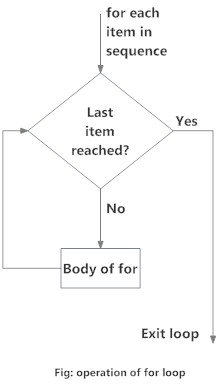# For Loop

### What is for loop in Python?

The for loop in Python is used to iterate over a sequence (list, tuple, string) or other iterable objects. Iterating over a sequence is called traversal.

#### Syntax of for Loop

for val in sequence:
# Body of for loop
Here, `val` is the variable that takes the value of the element inside the sequence on each iteration.
Loop continues until we reach the last item in the sequence. The body of for loop is separated from the rest of the code using indentation.

#### Flowchart of for Loop

Flowchart of for Loop in PythonFlowchart of for Loop in Python programming

#### Example: Python for Loop

# Program to find the sum of all numbers stored in a list
# List of numbers
numbers = [6, 5, 3, 8, 4, 2, 5, 4, 11]
# variable to store the sum
sum = 0
# iterate over the list
for number in numbers:
sum = sum + number
print("The sum is", sum)
When you run the program, the output will be:
The sum is 48

### The range() function

We can generate a sequence of numbers using `range()` function. `range(10)` will generate numbers from 0 to 9 (10 numbers).
We can also define the start, stop and step size as `range(start, stop, step_size)`. step_size defaults to 1 if not provided.
The `range` object is "lazy" in a sense because it doesn't generate every number that it "contains" when we create it. However, it is not an iterator since it supports `in`, `len` and `__getitem__` operations.
This function does not store all the values in memory; it would be inefficient. So it remembers the start, stop, step size and generates the next number on the go.
To force this function to output all the items, we can use the function `list()`.
The following example will clarify this.
print(range(10))
print(list(range(10)))
print(list(range(2, 8)))
print(list(range(2, 20, 3)))
Output
range(0, 10)
[0, 1, 2, 3, 4, 5, 6, 7, 8, 9]
[2, 3, 4, 5, 6, 7]
[2, 5, 8, 11, 14, 17]
We can use the `range()` function in `for` loops to iterate through a sequence of numbers. It can be combined with the `len()` function to iterate through a sequence using indexing. Here is an example.
# Program to iterate through a list using indexing
genre = ['pop', 'rock', 'jazz']
for g in genre:
print("I like", g)
# iterate over the list using index
for i in range(len(genre)):
print("I like", genre[i])
Output
I like pop
I like rock
​I like jazz

### for loop with else

A `for` loop can have an optional `else` block as well. The `else` part is executed if the items in the sequence used in for loop exhausts.
The `break` keyword can be used to stop a for loop. In such cases, the else part is ignored.
Hence, a for loop's else part runs if no break occurs.
Here is an example to illustrate this.
digits = [0, 1, 5]
for i in digits:
print(i)
else:
print("No elements left.")
When you run the program, the output will be:
0
1
5
No elements left.
Here, the for loop prints items of the list until the loop exhausts. When the for loop exhausts, it executes the block of code in the `else` and prints No items left.
This `for...else` statement can be used with the `break` keyword to run the `else` block only when the `break` keyword was not executed. Let's take an example:
# program to display people's ages from record
person_name = 'Daniel'
people = {'John': 45, 'Diana': 32, 'Artur': 90}
for person in people:
if person == person_name:
print(people[person])
break
else:
print('No entry with that name found.')
Output
No entry with that name found.